# Power Tips: Get to know LLC series resonant converter design

Resonant converters, especially the LLC series resonant converter (LLC-SRC) in Figure 1, have become popular over the last decade.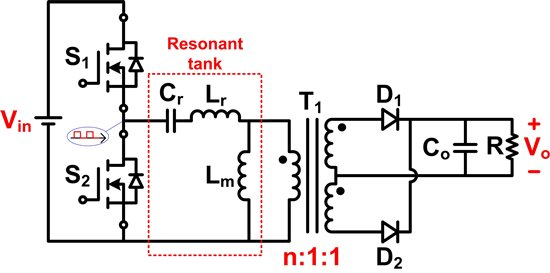Figure 1. Half-bridge LLC series resonant converter.

When compared to traditional isolated PWM converters, LLC-SRC  provides the following advantages:

• Lower component counts
• Higher converter efficiency
• Lower conduction/radiation electromagnetic interference (EMI)
• Low voltage stress on all circuit switches

All these benefits seem great, but when you realize its voltage gain equation is so complex you might think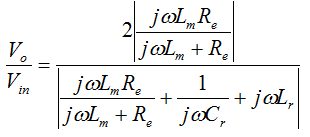“Wait a minute! There are more than two variables in the gain equation! I’m not going to do that.”

Don’t give up so fast. It only takes a few minutes to walk through this blog and understand how to play with LLC-SRC. You just need to figure out how to determine the transformer turns ratio and resonant tank parameters.

Let’s get over your fear of the voltage gain equation first. LLC-SRC has 50% duty cycles on its primary switches to generate a square wave at the input of its resonant tank. By applying sinusoidal approximation, LLC-SRC can be simplified to the following linear circuit: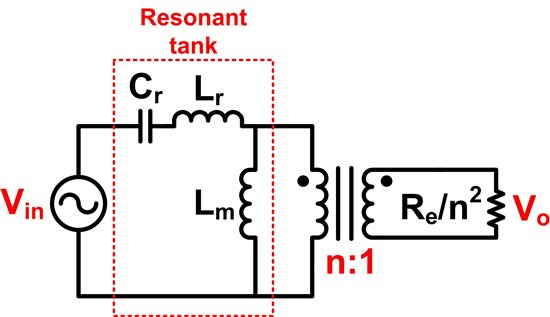Figure 2. Linearized LLC series resonant converter.

The voltage gain equation is derived from this linear circuit, but let’s ignore that “hard to memorize” equation for now. It’s notable that LLC-SRC efficiency is optimized when the switching frequency fs is equal to the resonant frequency fr. When fs=fr, the series connected LC becomes zero impedance, and the above linear circuit becomes Figure 3 below.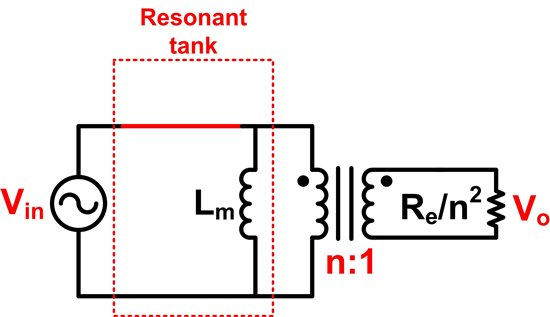Figure 3. Linearized LLC series resonant converter at fs=fr.

Input voltage and current are now in phase, and there is no reactive power dissipated in the circuit.  Now the input/output voltage gain becomes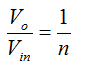In short, you just need to design your transformer turns ratio with the equation above at the input voltage you want your converter efficiency optimized.

When it comes to the resonant tank parameter (Lr, Lm, and Cs) design, many trade-offs need to be made. Let’s not confuse you with all these trade-offs. An easy way to start your resonant tank parameter design is to start with our LLC-SRC reference designs. For example, PMP5141 has Lr=72µH, Lm~350µH, and Cr=0.033 µF and is designed for 240W output power with 280V to 400V input voltage range. Alright, we get all the resonant tank details of a 240W LLC-SRC design. Where should we go from this point? It’s easy; just remember the following key tips for tuning up resonant tank parameters:

• Higher Lm/Lr ratio leads to a narrower regulation range (Input or output).
• Higher Lr/Cr ratio leads to worse load regulation and narrower regulation range.

If your LLC-SRC needs to operate in a wider input voltage range, you need a lower Lm/Lr ratio. If you are designing your LLC-SRC to function at a higher power level, you need a lower Lr/Cr ratio. Once you have your new resonant tank parameters, check them with the gain curve under different input voltages at full load to make sure the gain is high enough to provide the output voltage you need. The PMP5141 LLC-SRC gain curves below show that under 280V to 400V input voltages, I have enough gain to provide 24V output voltages at 240W load.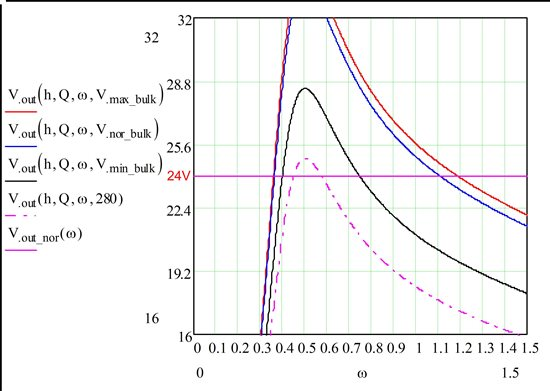See, it’s not that hard! Now you should feel more comfortable with a LLC-SRC design. Visit TI Designs to discover more LLC-SRC reference designs. Some LLC-SRC design examples are shown in the list below:

• PMP5327– Two stage PSU, 42V/6A output.
• PMP5967– 295VDC-400VDC Input, 12V/460W output.
• PMP8911 – Two stage PSU, 290VDC-400VDC Input at LLC-SRC, 56V/100W output.
• PMP8920 – Two stage PSU, 290VDC-400VDC Input at LLC-SRC, 12V/100W output.

• I'm designing a power supply with extended range, from 16 to 160Vdc, with an output of 24V@3A, when the input voltage is under 24V, the vout follow the vin. I have an asynchronous buck converter under test with good result, but at high voltage(vin over 90V) the inductor become very hot. Can an LLC converter work in my range?

• Ricky,

Use a voltage doubler could help on efficiency improvement and magnetic size reduction. PMP10283 design uses a voltage doubler at input which can handle the same output power as PMP9739 while using smaller size of magnetic.

I do think using a voltage doubler is the way to go in your case.

Aash,

You will need to have a voltage loop to control voltage and a current loop to control current. An example is PMP20946 reference design.

Regards,

Sheng-Yang Yu

• hi,

how to control output voltage and current individually?

Thankyou

• Hi,

For typical application where input is 53VAC to 90AVC (60Vpk to 132Vpk) and output is 43VDC (300W design) , i am trying to design single stage LLC HF Bridge topology using UCC25600.

After reading LLC related blogs and looking at input voltage variation I need to consider Lm/Lr low 3 ~ 4 (?). But input to output voltage conversion at low line input gives me transformer turns ratio n < 1 and at high line n > 1.

What could be the situation if n<1 and input is 90VAC (132Vpk)?

If I use voltage double input voltage range will be very high (144V) and need to compromise with efficiency.

Can any one suggest a possible way ?

Thanks

Rick